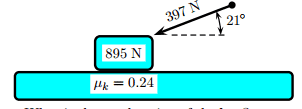# Find acceleration of a box given the forces on it...

## Homework Statement

A(n) 895 N crate is being pushed across a level floor by a force of 397 N at an angle of 21◦ above the horizontal. The coefficient of kinetic friction between the crate and the floor is 0.24. The acceleration of gravity is 9.81 m/s.What is the acceleration of the box? Answer in units of m/s/s

## The Attempt at a Solution

to find mass, Fg = mg
895 = m9.8
m = 91.3265

I've tried
ΣFy = FN - mg - FAsinθ
ΣFy = FN - mg - FAsinθ = ma
ΣFy = FN - mg - FAsinθ = 0
FN = mg + 397sin21
FN = 895 + 142.272
FN = 1037.27

∑Fx = FAcosθ - Ff
∑Fx = 397cos21 - (0.24)(1037.27)
397cos21 - (0.24)(1037.27) = ma
397cos21 - (0.24)(1037.27) = (91.3265)a
= 1.33 m/s/s but this is not correct

can anyone tell me where i went wrong/what is the correct way? thanks

## Answers and Replies

Simon Bridge
Science Advisor
Homework Helper
Note: ##\sum\vec F = m\vec a = (mg)\vec a/g \implies \vec a/g = \frac{1}{mg}\sum\vec F## ... ie. the net force divided by the weight is the acceleration in gees.
ie. your answer would have been a = 0.14g. Then, no need to do that 1st division risking a possible rounding error.

I bring this up because your reasoning seems fine - so the error will be in arithmetic or rounding off or something like that... or a mistake in the problem itself.

So redo from the start...
Info:
W=895N, F=397N, ##\theta##=21deg, ##\mu##=0.24
... the task is to derive an equation for the acceleration in terms of just these 4 things.

Off free body diagram ##\sum\vec F = m\vec a## :
(1) ##F\cos\theta - \mu N = ma = (W/g)a## since ##W=mg##
(2) ##N-W-F\sin\theta = 0##
(N=normal force)

Do all the algebra first - when you have derived the final equatio, then you can put the numerical values in.

gneill
Mentor
The problem describes the applied force as having an angle of 21° above the horizontal, but the diagram seems to show it as directed below the horizontal. Could it be that the diagram is not right?

•Simon Bridge